Home
Hostname: page-component-568f69f84b-jtg5s Total loading time: 0.216 Render date: 2021-09-20T03:35:56.821Z Has data issue: true Feature Flags: { "shouldUseShareProductTool": true, "shouldUseHypothesis": true, "isUnsiloEnabled": true, "metricsAbstractViews": false, "figures": true, "newCiteModal": false, "newCitedByModal": true, "newEcommerce": true, "newUsageEvents": true }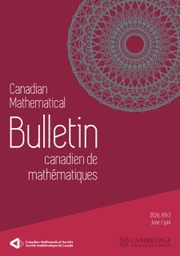Canadian Mathematical Bulletin

# The Saddle-Point Method and the Li Coefficients

Published online by Cambridge University Press:  20 November 2018

## Abstract

HTML view is not available for this content. However, as you have access to this content, a full PDF is available via the ‘Save PDF’ action button.

In this paper, we apply the saddle-point method in conjunction with the theory of the Nörlund–Rice integrals to derive precise asymptotic formula for the generalized Li coefficients established by Omar and Mazhouda. Actually, for any function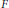$F$ in the Selberg class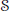$\mathcal{S}$ and under the Generalized Riemann Hypothesis, we have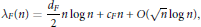$${{\lambda }_{F}}(n)\,=\,\frac{{{d}_{F}}}{2}n\,\log \,n\,+\,{{c}_{F}}n\,+\,O(\sqrt{n}\,\log \,n),$$

with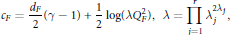$${{c}_{F}}\,=\,\frac{{{d}_{F}}}{2}(\gamma \,-\,1)\,+\,\frac{1}{2}\log (\lambda \text{Q}_{F}^{2}),\,\,\lambda \,=\,\prod\limits_{j=1}^{r}{\lambda _{j}^{2{{\lambda }_{j}}}},$$

where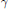$\gamma$ is the Euler's constant and the notation is as below.

## Keywords

Type
Research Article
Information
Canadian Mathematical Bulletin , 01 June 2011 , pp. 316 - 329

## References

 Bombieri, E. and Hejhal, D. A., On the distribution of zeros of linear combinations of Euler products. Duke. Math. J. 80(1995), no. 3, 821862. doi:10.1215/S0012-7094-95-08028-4CrossRefGoogle Scholar
 Bombieri, E. and Lagarias, J. C., Complements to Li's criterion for the Riemann hypothesis. J. Number Theory 77(1999), no. 2, 274287. doi:10.1006/jnth.1999.2392CrossRefGoogle Scholar
 Coffey, M. W., Relations and positivity results for the derivatives of the Riemann ξ function. J. Comput. Appl. Math. 166(2004), no. 2, 525534. doi:10.1016/j.cam.2003.09.003CrossRefGoogle Scholar
 Coffey, M. W., Toward verification of the Riemann hypothesis. Math. Phys. Anal. Geom. 8(2005), no. 3, 211255. doi:10.1007/s11040-005-7584-9CrossRefGoogle Scholar
 Flajolet, P. and Vepstas, L., On differences of zeta values. J. Comput. Appl. Math. 220(2008), no. 1–2, 5873. doi:10.1016/j.cam.2007.07.040CrossRefGoogle Scholar
 Kaczorowski, J. and Perelli, A., The Selberg class: a survey. In: Number theory in progress, 2, de Gruyter, Berlin, 1999, pp. 953992.Google Scholar
 Lagarias, J. C., Li coefficients for automorphic L-functions. Ann. Inst. Fourier 57(2007), no. 5, 16891740.CrossRefGoogle Scholar
 Li, X.-J., The positivity of a sequence of number and the Riemann hypothesis. J. Number Theory 65(1997), no. 2, 325333. doi:10.1006/jnth.1997.2137CrossRefGoogle Scholar
 Li, X.-J., Explicit formulas for Dirichlet and Hecke L-functions. Illinois J. Math. 48(2004), no. 2, 491503.CrossRefGoogle Scholar
 Maslanka, K., Effective method of computing Li's coefficients and their properties. Experimental Math., to appear.Google Scholar
 Omar, S. and Mazhouda, K., Le critère de Li et l’hypothèse de Riemann pour la classe de Selberg. J. Number Theory 125(2007), no. 1, 5058. doi:10.1016/j.jnt.2006.09.013CrossRefGoogle Scholar
 Omar, S. and Mazhouda, K., Le critère de positivité de Li pour la classe de Selberg. C. R. Acad. Sci. Paris 345(2007), no. 5, 245248.CrossRefGoogle Scholar
 Omar, S. and Mazhouda, K., Corrigendum et addendum à Le critère de Li et l’hypothèse de Riemann pour la classe de Selberg [J. Number Theory 125(2007), no. 1, 50–58]. J. Number Theory 130(2010), no. 4, 11091114. doi:10.1016/j.jnt.2009.10.010CrossRefGoogle Scholar
 Rudnick, Z. and Sarnak, P., Zeros of principal L-functions and radom matrix theory. Duke. Math. J. 81(1996), no. 2, 269322. doi:10.1215/S0012-7094-96-08115-6CrossRefGoogle Scholar
 Selberg, A., Old and new conjectures and results about a class of Dirichlet series. In: Proceedings of the Amalfi conference on analytic number theory (Maiori, 1989), Univ. Salermo, 1992, pp. 367385.Google Scholar
 Srinivas, K., Distinct zeros of functions in the Selberg class. Acta. Arith. 103(2002), no. 3, 201207. doi:10.4064/aa103-3-1CrossRefGoogle Scholar
 Voros, A., A sharpening of Li's criterion for the Riemann hypothesis. Math. Phys. Anal. Geom. 9(2006), no. 1, 5363. doi:10.1007/s11040-005-9002-8CrossRefGoogle ScholarYou have Access
2
Cited by

# Send article to Kindle

Note you can select to send to either the @free.kindle.com or @kindle.com variations. ‘@free.kindle.com’ emails are free but can only be sent to your device when it is connected to wi-fi. ‘@kindle.com’ emails can be delivered even when you are not connected to wi-fi, but note that service fees apply.

Find out more about the Kindle Personal Document Service.

The Saddle-Point Method and the Li Coefficients
Available formats
×

# Send article to Dropbox

To send this article to your Dropbox account, please select one or more formats and confirm that you agree to abide by our usage policies. If this is the first time you use this feature, you will be asked to authorise Cambridge Core to connect with your <service> account. Find out more about sending content to Dropbox.

The Saddle-Point Method and the Li Coefficients
Available formats
×

# Send article to Google Drive

To send this article to your Google Drive account, please select one or more formats and confirm that you agree to abide by our usage policies. If this is the first time you use this feature, you will be asked to authorise Cambridge Core to connect with your <service> account. Find out more about sending content to Google Drive.

The Saddle-Point Method and the Li Coefficients
Available formats
×
×